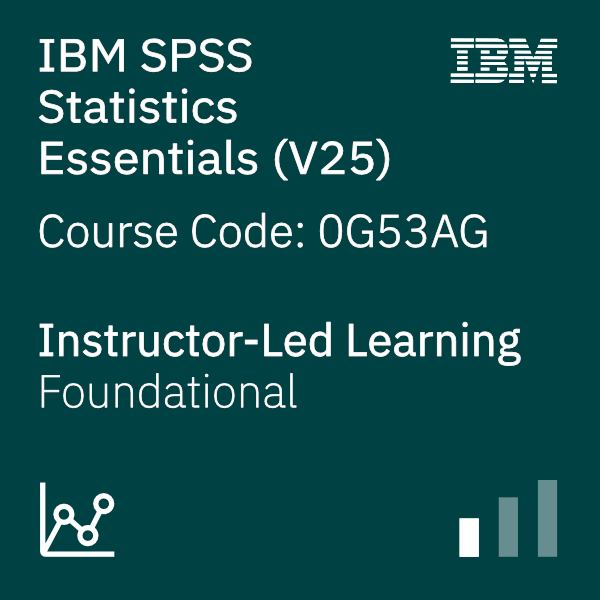# Overview

This course guides students through the fundamentals of using IBM SPSS Statistics for typical data analysis process. Students will learn the basics of reading data, data definition, data modification, and data analysis and presentation of analytical results. Students will also see how easy it is to get data into IBM SPSS Statistics so that they can focus on analyzing the information. In addition to the fundamentals, students will learn shortcuts that will help them save time. This course uses the IBM SPSS Statistics Base features.

# Audience

• Anyone with little or no experience in using IBM SPSS Statistics.
• Anyone who is new to using a statistical package for data analysis.
• Anyone who is considering purchasing IBM SPSS Statistics.

# Prerequisites

• Experience with IBM SPSS Statistics is not necessary, though a basic understanding of purpose and functions of the software is helpful.
• No statistical background is necessary.

# Objective

• Overview of IBM SPSS Statistics
• Read data and define metadata
• Transform variables
• Use functions to compute variables
• Set the unit of analysis
• Merge data files
• Select cases for analyses
• Summarize individual variables
• Describe relationships between variables
• Create presentation-ready tables with Custom Tables
• Customize pivot tables
• Create and customize charts
• Work with syntax
• Control the IBM SPSS Statistics environment
Show details

# Course Outline

Introduction to IBM SPSS Statistics
• Review basic concepts in IBM SPSS Statistics
• Identify the steps in the research process
• Review basic analyses
• Use Help

Read data and define metadata
• Overview of data sources
• Read data from databases
• Read data from Microsoft Excel
• Define variable properties

Transform variables
• Compute new variables
• Recode values of nominal, ordinal, and scale variables
• Create a numeric variable from a string variable
• Explore advanced variable transformations

Use functions to compute variables
• Use statistical functions
• Use logical functions
• Use missing value functions
• Use conversion functions
• Use system variables
• Use the Data and Time Wizard

Set the unit of analysis
• Remove duplicate cases
• Create aggregated datasets
• Restructure datasets

Merge data files
• Add cases from one dataset to another
• Add variables from one dataset to another
• Enrich a dataset with aggregated information

Select cases for analyses
• Select case for an analysis
• Run analyses for subgroups

Summarize individual variables
• Define levels of measurement
• Summarizing categorical variables with Frequencies
• Summarizing scale variables with Frequencies and Descriptives

Describe relationship between variables
• Select the appropriate procedure to summarize the relationship between two variables
• Summarize the relationship between categorical variables with Crosstabs
• Summarize the relationship between a scale and a categorical variable with Means

Create presentation-ready tables with Custom Tables
• Identify table layouts
• Create tables for variables with shared categorical
• Create tables for variables that represent a multiple response question

Customize pivot tables
• Perform Automated Output Modification
• Customize pivot tables in the Pivot Table Editor
• Use table templates
• Export pivot tables to other applications

Create and customize charts
• Use the Chart Builder to create various types of graphs
• Customize charts in the Chart Editor
• Use chart templates
• Export pivot tables to other applications

Work with syntax
• Use syntax to automate analyses
• Use the Syntax Editor environment
• Create and edit syntax
• Run syntax

Control the IBM SPSS Statistics environment
• Describe the features of the Options dialog box
• Reset the display of variables
• Set the default working data folders